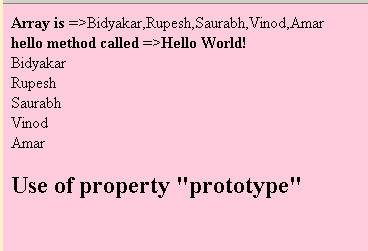Tutorials

# JavaScript array prototype

We can add and modify the properties and methods of the array by using the property "prototype" of the array class.

We can add and modify the properties and methods of the array by using the property "prototype" of the array class.

# JavaScript array prototype

We can add and modify the properties and methods of the array by using the property "prototype" of the array class. In the web developing application we might require some times additional functionality rather than having the predefined methods like push(), pop(), reverse() etc. In this situation the property "prototype" is quite useful for developers. We can create new methods or functions and can modify the existing functions by the use of "prototype" property.

Below we have shown an example into which we have created few new methods which can be called by using the array object. Here is the full example code for this prototype example as follows:

javascript_array_prototype.html

 JavaScript array prototype example

Use of property "prototype"

Output of the example is as given below: